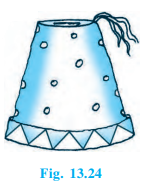# A fez, the cap used by the Turks, is shaped like the frustum of a cone (see Fig.) . If its radius on the open side is 10 cm, radius at the upper base is 4 cm and its slant height is 15 cm, find the area of material used for making it.Given

Slant height of frustum (l)= 15 cm

Let radius of smaller circle end (r) = 4 cm

Let radius of larger circle end (R) = 10 cm

Find out

We have to find the area of material used for making it

Solution

Area of material used for making it = Curve surface area + area of upper base

= (π(r+R)l) + (πr2)

= π ((r+R)l + r2)

π ((4+10) × 15 + (4 × 4))= 22/7× (226)

= 710.286 cm2

Hence, the area of material used for making it = 710.286 cm(1)(0)